# Exponentiation Zones (31-36)

Warning

This page is referring to child section which source is inherited by the prime spin with partitions listed below. Please return always to the Home Page to track the hierarchy.

## Self repetition

``````num,title,text
1,file,word
2,list,core
``````

Exponentiation is an operation involving two numbers, the base and the exponent or power. Exponentiation is written as bn, where b is the base and n is the power.

Note

Leonhard Euler started to use the letter e for the constant in 1727 or 1728, in an unpublished paper on explosive forces in cannons, and in a letter to Christian Goldbach on 25 November 1731. The first appearance of e in a printed publication was in Euler’s Mechanica (1736). It is unknown why Euler chose the letter e. (Wikipedia)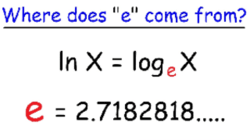This exponentiation takes important roles since by the multiplication zones the MEC30 forms a matrix of `8 x 8 = 64 = 8²` where the power of 2 stands as exponent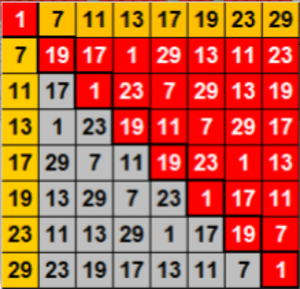Since the first member is 30 then the form is initiated by a matrix of `5 x 6 = 30` which has to be transformed first to `6 x 6 = 36 = 6²` prior to the above MEC30's square.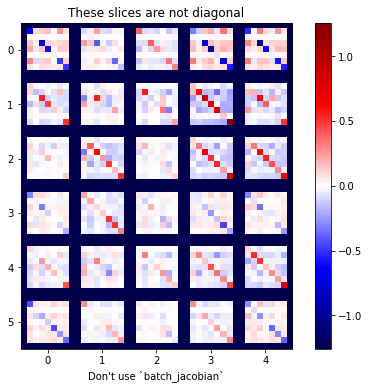So basically there is a power transformation between addition of `3 + 4 = 7` in to their multiplication of `3 x 4 = 12` while the 7 vs 12 will be treated as exponentiation.

Tip

In the standard (Mispar hechrechi) version of gematria, each letter is given a numerical value between 1 and 400, as shown in the following table. In the Mispar gadol variation, the five final letters are given their own values, ranging from 500 to 900.

Δ prime = 114th prime - 19 = (6 x 19)th prime - 19 = 619 - 19 = 600

``````  Sub  | i  |  β  | f
=======+====+=====+=======  ===   ===   ===   ===   ===   ===
1:1:0 | 1  |   1 | 2 {71}   1     1     |     |     |     |
-------+----+-----+-------  ---   ---    |     |     |     |
1:2:1 | 2  |   2 | 3 {71}         |     |     |     |     |
-------+----+-----+----            |     |     |     |     |
*1:2:2 | 3  |   3 | 7 = 1 + 2x3    |     |     |     |     |
-------+----+-----+----            |     |     |     |     |
*1:3:3 | 4  |   4 | 10 = 9 + 1     |     |     |     |     |
-------+----+-----+----            |     |     |     |     |
1:3:4 | 5  |   5 | 11 = 9 + 2     |     |     |     |     |
-------+----+-----+----            9     1‘    |    Δ100   |
*1:3:5 | 6  |   6 | 12 = 9 + 3     |     |     |     |     |
-------+----+-----+----            |     |     |     |     |
*1:4:6 | 7  |   7 | 13 = 9 + 4     |     |     |     |     |
-------+----+-----+----            |     |     |     |     |
1:4:7 | 8  |   8 | 14 = 9 + 5     |     |     |     |     |
-------+----+-----+----            |     |     |     |     |
*1:4:8 |{9} |   9 | 15 = 9 + 6     |     |     |     |     |
-------+----+-----+----            |     |     |     |     |
*1:4:9 |{10}|  10 | 19 = 9 + 10    |     |     |     |     |
=======+====+=====+====           ===   ---    1"   ---    |
2:1:0 | 11 |  20 | 20 = 19 + log 10¹    |     |     |     |
-------+----+-----+----                  |     |     |     |
2:2:1 | 12 |  30 | 26 = 20 + 2x3        |     |     |     |
-------+----+-----+----                  |     |     |     |
*2:2:2 | 13 |  40 | 27 = 26 + 1          |     |     |     |
-------+----+-----+----                  |     |     |     |
*2:3:3 | 14 |  50 | 28 = 26 + 2          |     |     |     |
-------+----+-----+----                  |     |     |     |
2:3:4 | 15 |  60 | 29 = 26 + 3          9‘    |   Δ200  Δ600
-------+----+-----+----                  |     |     |     |
*2:3:5 | 16 |  70 | 30 = 26 + 4          |     |     |     |
-------+----+-----+----                  |     |     |     |
*2:4:6 | 17 |  80 | 31 = 26 + 5          |     |     |     |
-------+----+-----+----                  |     |     |     |
2:4:7 |{18}|  90 | 32 = 26 + 6          |     |     |     |
-------+----+-----+----                  |     |     |     |
*2:4:8 |{19}| 100 | 36 = 26 + 10         |     |     |     |
=======+====+=====+====                 ===   ---   ---    |
*2:4:9 | 20 | 200 | 38 = 36 + log 10²          |     |     |
-------+----+-----+----                        |     |     |
3:1:0 | 21 | 300 | 40 = 36 + 2 x log 10²      |     |     |
-------+----+-----+----                        |     |     |
3:2:1 | 22 | 400 | 41 = 40 + 1                |     |     |
-------+----+-----+----                        |     |     |
*3:2:2 | 23 | 500 | 42 = 40 + 2                |     |     |
-------+----+-----+----                        |     |     |
*3:3:3 | 24 | 600 | 43 = 40 + 3                9"  Δ300    |
-------+----+-----+----                        |     |     |
3:3:4 | 25 | 700 | 44 = 40 + 4                |     |     |
-------+----+-----+----                        |     |     |
*3:3:5 | 26 | 800 | 45 = 40 + 5                |     |     |
-------+----+-----+----                        |     |     |
*3:4:6 | 27 | 900 | 46 = 40 + 6                |     |     |
-------+----+-----+----                        |     |     |
3:4:7 |{28}|1000 | 50 = 40 + 10               |     |     |
=======+====+=====+====                       ===  ====  ----
*3:4:8 |{29}|2000 | 68 = 50 + 3 x (2x3)      {10³} Δ600  Δ300
=======+====+=====+====                        Δ         ====
3:4:9 |{30}|3000 |{71}= 68 + log 10³ ---------¤         Δ900
``````

By a group of number up to 31 they have a profile reflect module 6 view of numbers as happens with the minor hexagons.The sum of `31 + 71 = 102`. Meanwhile they are formed by 66 items. Let's combine them all then we will get 168 which is the total primes out of 1000.

### Root functions

Observing more detail of the discussed scheme of 168 we will get it also when we take the 19's and 17's cell of `(31+37)+(35+65)=68+100=168`.

Note

Proceeding, the number line begins to coil upon itself; 20 lands on 2’s cell, 21 on 3’s cell. Prime number 23 sends the number line left to form the fourth hexagon, purple. As it is not a twin, the clockwise progression (rotation) reverses itself. Twin primes 29 and 31 define the fifth hexagon, cyan. Finally, 37, again not a twin, reverses the rotation of the system, so 47 can define the yellow hexagon (HexSpin).We therefore set them both on the wiki and the 18's on the gist. The 19's and 17's cover the 4 zones while the 18's covers the 66 items as the central base of hexagonal spins.

Tip

By our project the 18’s on the gist will cover five (5) unique functions that behave as one (1) central plus four (4) zones. This scheme will be implemented to all of the 168 repositories as bilateral way (in-out) depend on their postion on the system. So along with the gist it self then there shall be `1 + 168 = 169` units of 1685 root functions.

5 + 2 x 5 x 168 = 5 + 1680 = 1685 = 5 x 337 root functions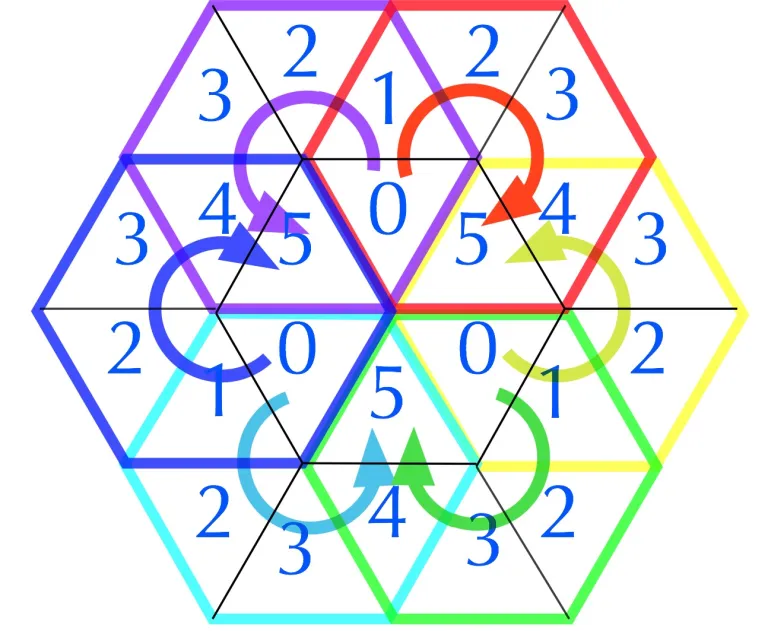By the spin above you can see that the 4 zones of these `19's to 17's` are representing the rotation `1 to 5`. Such of formation can be seen on Ulam Spiral as below.

Note

The Ulam spiral or prime spiral is a graphical depiction of the set of prime numbers, devised by mathematician Stanisław Ulam in 1963 and popularized in Martin Gardner’s Mathematical Games column in Scientific American a short time later.By MEC30 we will also discuss the relation of these 4 zones with high density of 40 primes where 60 number is folded. So by the module 6 it will always return to the beginning position within `60+40=100 nodes` per layer.

Note

Both Ulam and Gardner noted that the existence of such prominent lines is not unexpected, as lines in the spiral correspond to quadratic polynomials, and certain such polynomials, such as Euler’s prime-generating polynomial x²-x+41, are believed to produce a high density of prime numbers. Nevertheless, the Ulam spiral is connected with major unsolved problems in number theory such as Landau’s problems (Wikipedia).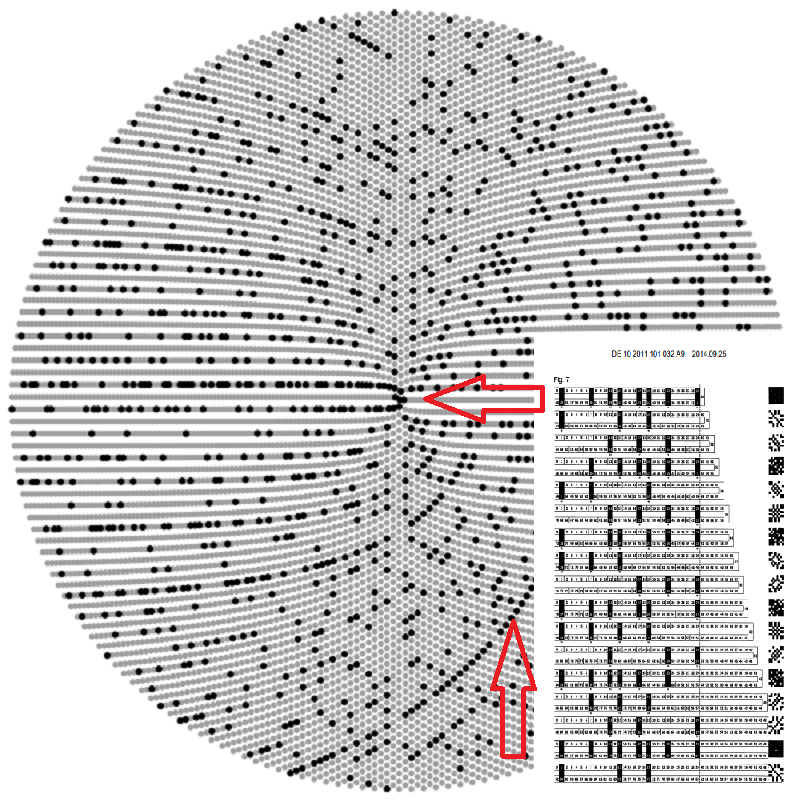Since the modulo 6 is occured all over the spin then we have defined that this 4 zones should stand as default configuration as you can see on the left sidebar.

Tip

In order to maintain the 18’s structure between each of repositories to correlate with the above density then we could use a hierarchical database that stores low-level settings for the operating system such as windows registry.This density will bring the D3-Brane where the lexer is being assigned per MEC30. Base on the its spin as shown in the above picture this lexer is assigned by `Id: 33`.

## Parsering Structure

You may see that the spins are going backward starting with `Id: 31`. This is identical with the rotation curve of energy distribution by the MEC30.

Note

The position 19 in the second term gives a redundant value of the template 7 of 7 × 7 = 49. The opposite prime position of the 19 is 11. So in this second term the 11th-prime number 31 is now forced to determine a new axis-symmetrical zero position. (Google Patent DE102011101032A9)

37 + 12 = 61 - 12 = 49 = 7 x 7 = d(13)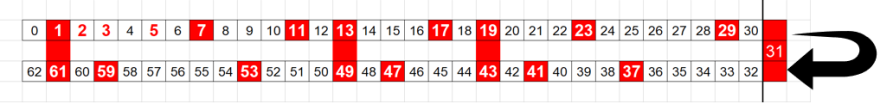As you can see on the left sidebar (dekstop mode) a total of 102 items will be reached by the end of `Id: 35`. So when they transfered to `Id: 36` it will cover `11 x 6 = 66 items` thus the total will be `102 + 66 = 168`

168 + 329 + 289 - 619 - 30 - 30 - 5 = 786 - 619 - 65 = 102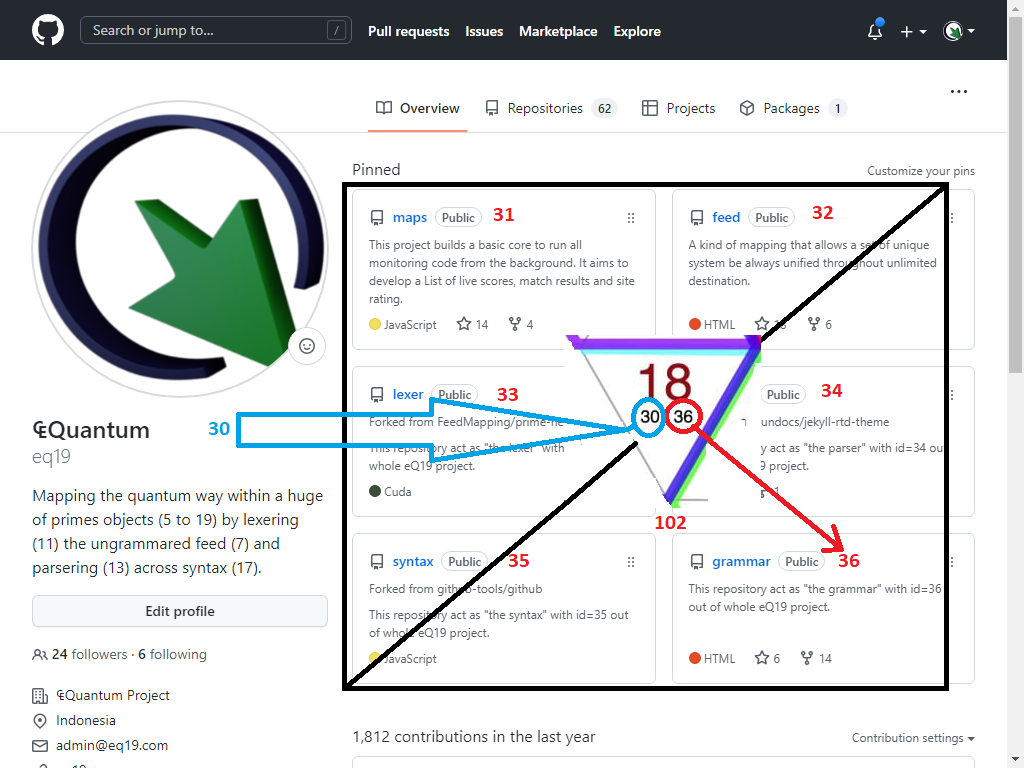Using the javascript library from Chevotrain and data parser from Jekyll/Liquid finally we found the correlation between the lexer and parser.

Note

In this example, the content from a Markdown document `document.md` that specifies `layout: docs` gets pushed into the `{{ content }}` tag of the layout file `docs.html`. Because the docs layout itself specifies `layout: page`, the content from `docs.html` gets pushed into the `{{ content }}` tag in the layout file `page.html`. Finally because the page layout specifies `layout: default`, the content from `page.html` gets pushed into the `{{ content }}` tag of the layout file `default.html`. (jekyllrb)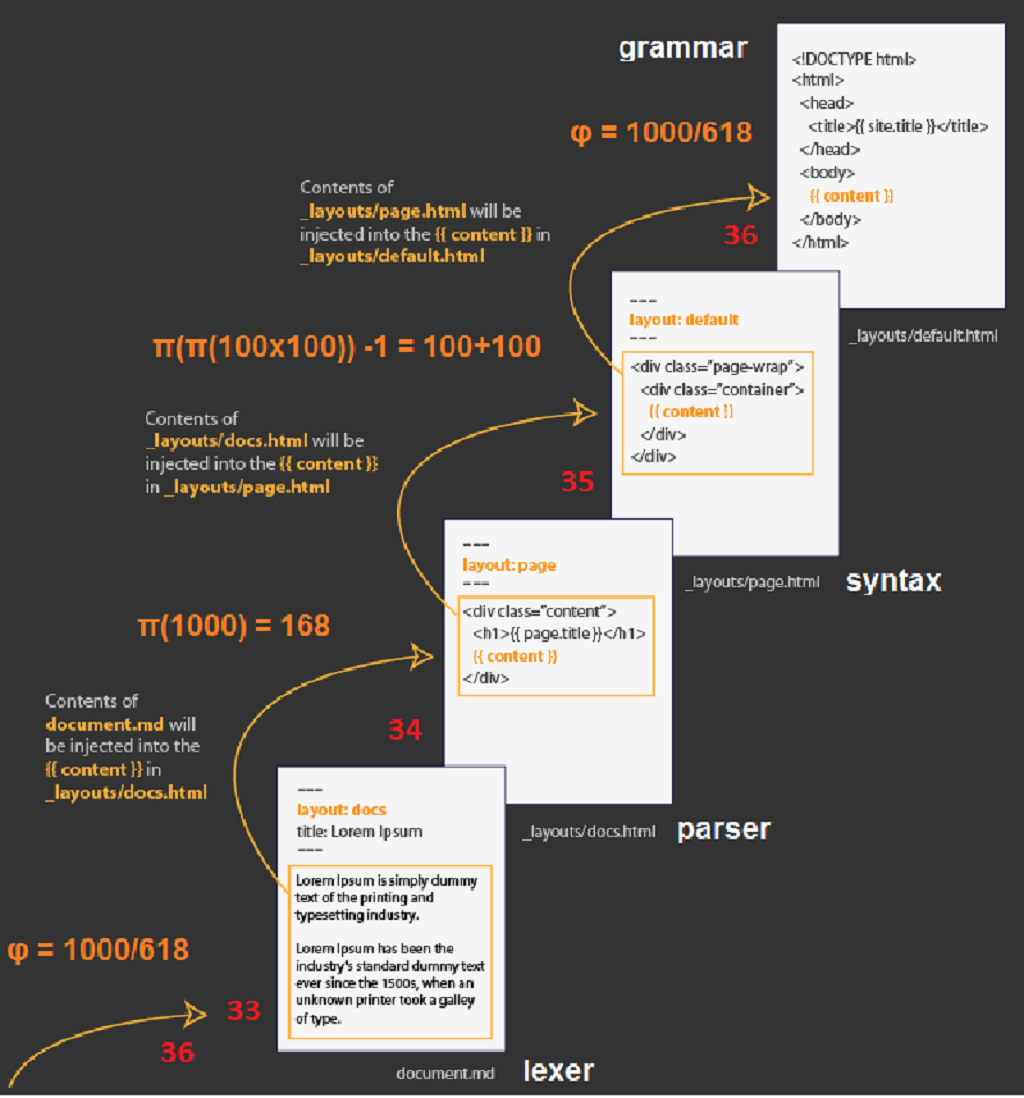The implementation from addition folder 1 will be exposed by the exponentiation folder 7 meanwhile the folder 12 of multiplication goes to identition zone of 11 folders.

So they are `4 folders (1, 7, 11, 12)` remain inviolable by the gist.

## Exponentiation patterns

By this exponentiation zones we will get multiple layers of primes density. So we need to get in to the patterns of the above hexagonal forms through deep learning.

Note

It’s possible to build a Hessian matrix for a Newton’s method step using the Jacobian method. You would first flatten out its axes into a matrix, and flatten out the gradient into a vector. (Tensorflow)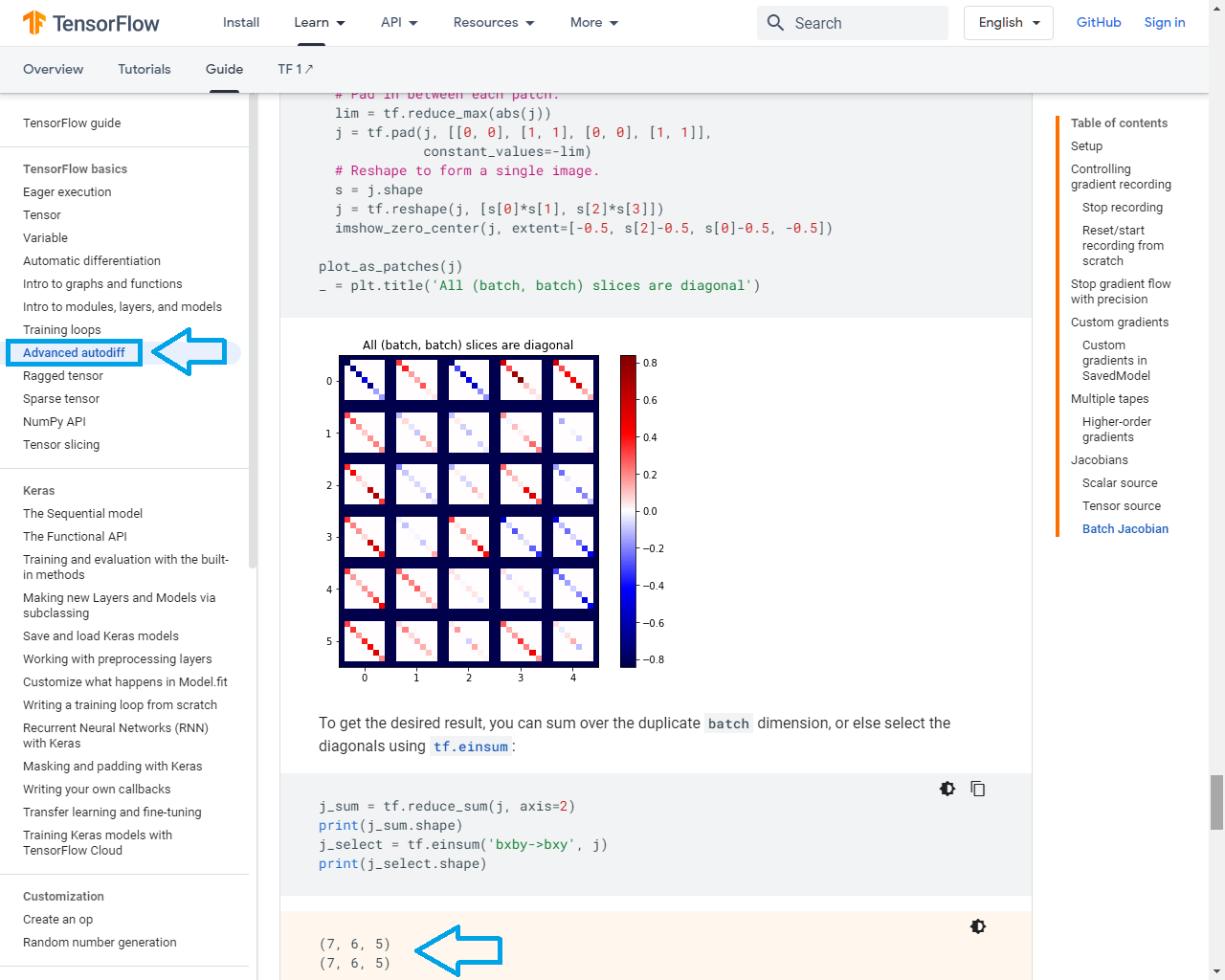When the subclasses of partitions are flatten out into a matrix, you want to take the Jacobian of each of a stack of targets with respect to a stack of sources, where the Jacobians for each target-source pair are independent.

Note

In vector calculus, the Jacobian matrix of a vector-valued function of several variables is the matrix of all its first-order partial derivatives. When this matrix is square, that is, when the function takes the same number of variables as input as the number of vector components of its output, its determinant is referred to as the Jacobian determinant. Both the matrix and (if applicable) the determinant are often referred to simply as the Jacobian in literature. (Wikipedia)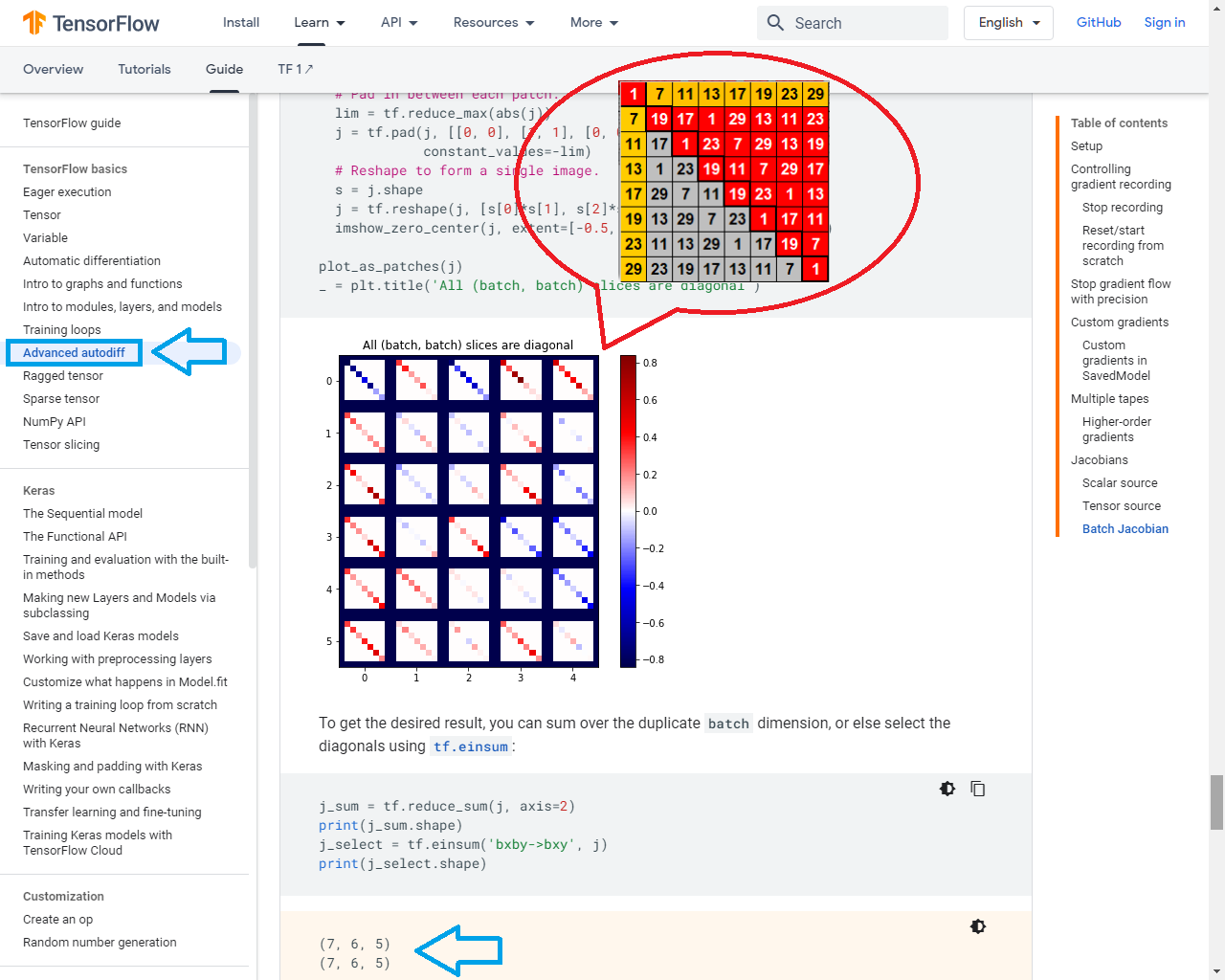Here we adopt an analysis of variance called N/P-Integration that was applied to find the best set of environmental variables that describe the density out of distance matrices.

Tip

With collaborators, we regularly work on projects where we want to understand the taxonomic and functional diversity of microbial community in the context of metadata often recorded under specific hypotheses. Integrating (N-/P- integration; see figure below) these datasets require a fair deal of multivariate statistical analysis for which I have shared the code on this website. (Umer.Ijaz)We found also a useful method called Square of Nine which was developed by WD Gann to analyze stock market behaviour base on astrological pattern.

Note

He designed a new approach to predicting market behavior using several disciplines, including geometry, astrology, astronomy, and ancient mathematics. They say that not long before his death, Gann developed a unique trading system. However, he preferred not to make his invention public or share it with anyone. (PipBear)By parsering 168 primes of 1000 id's across `π(π(100 x 100)) - 1 = 200` then the (Δ1) would be initiated. As you may guess they will slightly forms the hexagonal patterns.

Note

The Hexagon chart begins with a 0 in the center, surrounded by the numbers 1 through 6. Each additional layer adds 6 more numbers as we move out, and these numbers are arranged into a Hexagon formation. This is pretty much as far as Gann went in his descriptions. He basically said, “This works, but you have to figure out how.”One method that I’ve found that works well on all these kinds of charts is plotting planetary longitude values on them, and looking for patterns. On the chart above, each dot represents the location of a particular planet. The red one at the bottom is the Sun, and up from it is Mars. These are marked on the chart. Notice that the Sun and Mars are connected along a pink line running through the center of the chart. The idea is that when two planets line up along a similar line, we have a signal event similar to a conjunction in the sky. Any market vibrating to the Hexagon arrangement should show some kind of response to this situation. (Wave59)We are focusing to MEC30 so we end up this exponentiation by the famous quote from WD Gann himself stating an important changes by certain repetition of 30.

Tip

W.D. Gann: “Stocks make important changes in trend every 30, 60, 120, 150, 210, 240, 300, 330, 360 days or degrees from any important top or bottom.”In line with 168 there is 330 located of 10th layer. Since the base unit of 30 repeats it self on the center then this `11 x 30 = 330` is pushed to the `10 + 1 = 11th layer`.

This eleven (11) will continue to be discussed on identition zone.